Balbharti Maharashtra State Board 11th Maths Book Solutions Pdf Chapter 4 Methods of Induction and Binomial Theorem Ex 4.3 Questions and Answers.

## Maharashtra State Board 11th Maths Solutions Chapter 4 Methods of Induction and Binomial Theorem Ex 4.3

Question 1.
In the following expansions, find the indicated term.
(i) $$\left(2 x^{2}+\frac{3}{2 x}\right)^{8}$$, 3rd term
Solution: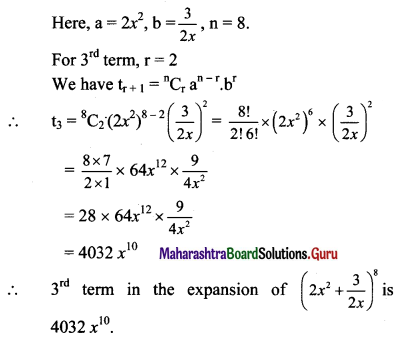(ii) $$\left(x^{2}-\frac{4}{x^{3}}\right)^{11}$$, 5th term
Solution: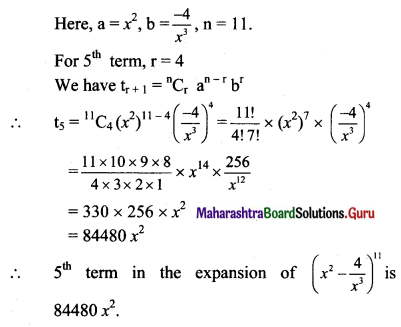(iii) $$\left(\frac{4 x}{5}-\frac{5}{2 x}\right)^{9}$$, 7th term
Solution: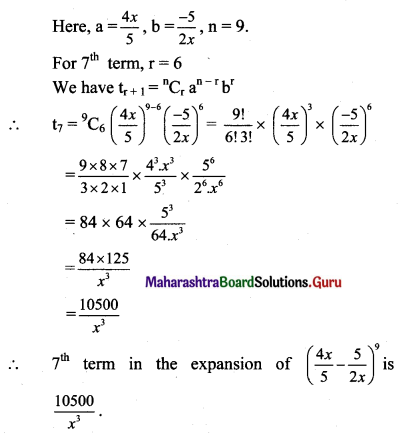(iv) In $$\left(\frac{1}{3}+a^{2}\right)^{12}$$, 9th term
Solution: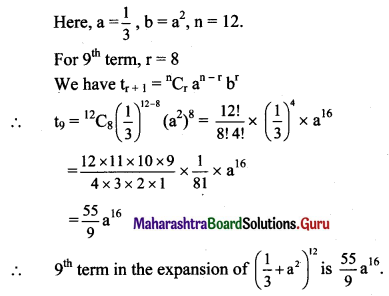(v) In $$\left(3 a+\frac{4}{a}\right)^{13}$$, 10th term
Solution: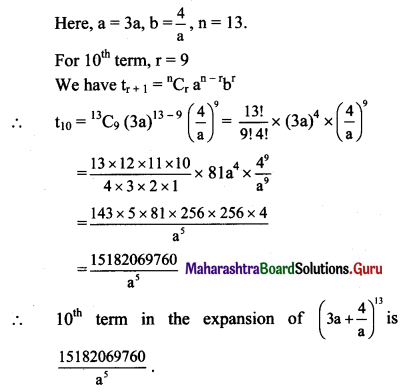Question 2.
In the following expansions, find the indicated coefficients.
(i) x3 in $$\left(x^{2}+\frac{3 \sqrt{2}}{x}\right)^{9}$$
Solution: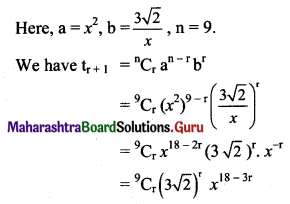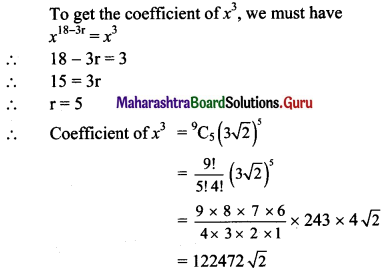(ii) x8 in $$\left(2 x^{5}-\frac{5}{x^{3}}\right)^{8}$$
Solution: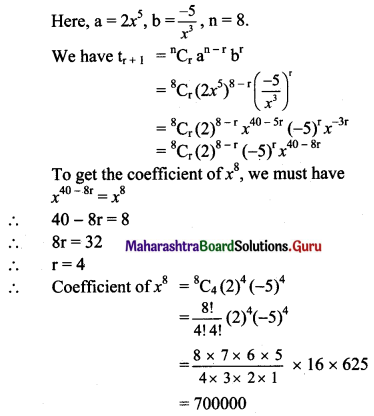(iii) x9 in $$\left(\frac{1}{x}+x^{2}\right)^{18}$$
Solution: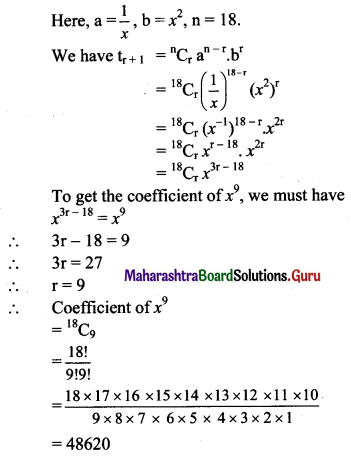(iv) x-3 in $$\left(x-\frac{1}{2 x}\right)^{5}$$
Solution: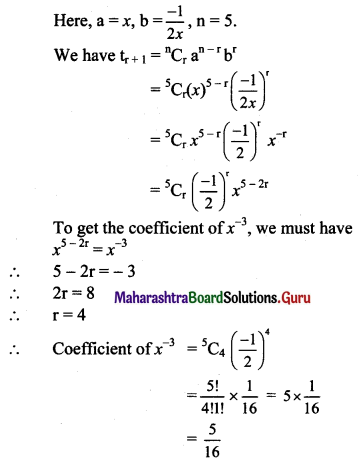(v) x-20 in $$\left(x^{3}-\frac{1}{2 x^{2}}\right)^{15}$$
Solution: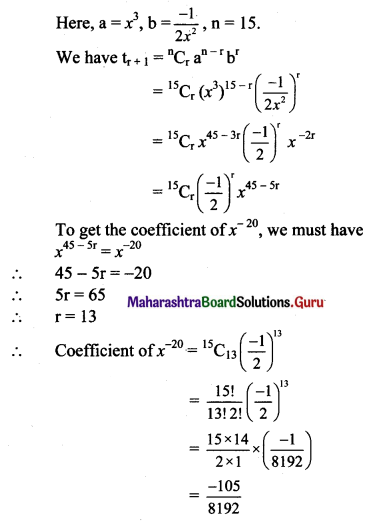Question 3.
Find the constant term (term independent of x) in the expansion of
(i) $$\left(2 x+\frac{1}{3 x^{2}}\right)^{9}$$
Solution: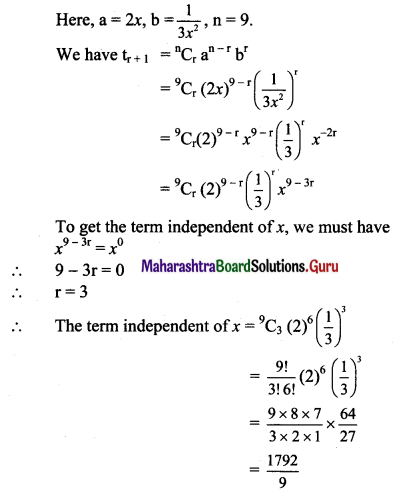(ii) $$\left(x-\frac{2}{x^{2}}\right)^{15}$$
Solution: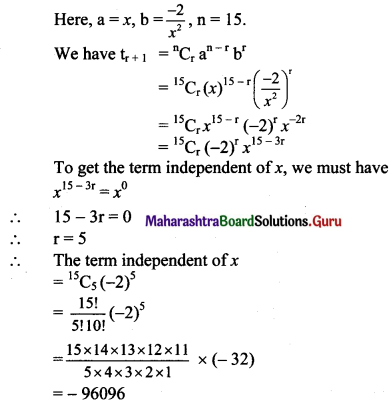(iii) $$\left(\sqrt{x}-\frac{3}{x^{2}}\right)^{10}$$
Solution: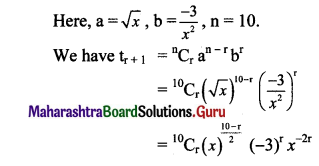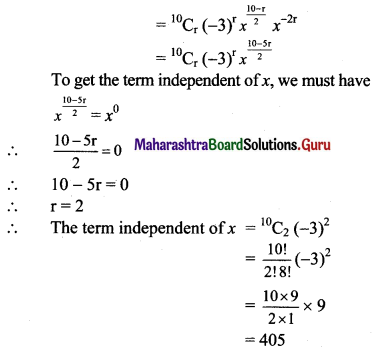(iv) $$\left(x^{2}-\frac{1}{x}\right)^{9}$$
Solution: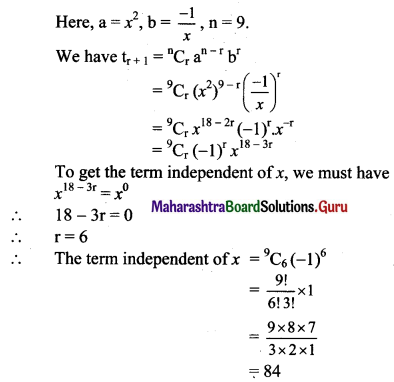(v) $$\left(2 x^{2}-\frac{5}{x}\right)^{9}$$
Solution: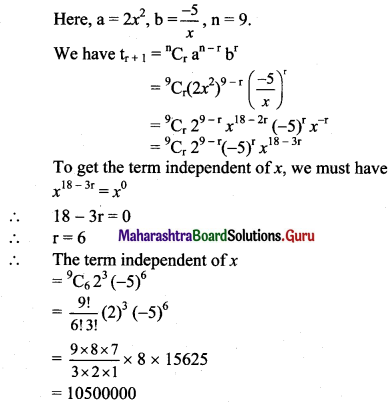Question 4.
Find the middle terms in the expansion of
(i) $$\left(\frac{x}{y}+\frac{y}{x}\right)^{12}$$
Solution: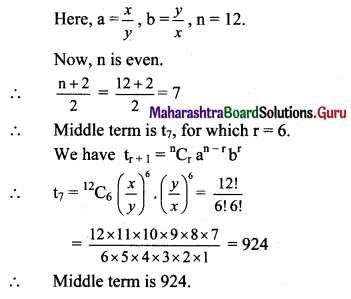(ii) $$\left(x^{2}+\frac{1}{x}\right)^{7}$$
Solution: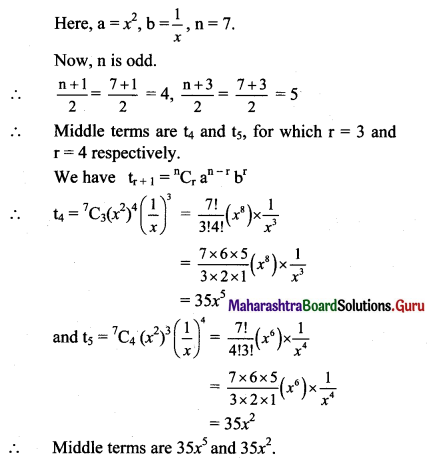(iii) $$\left(x^{2}-\frac{2}{x}\right)^{8}$$
Solution: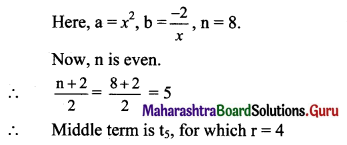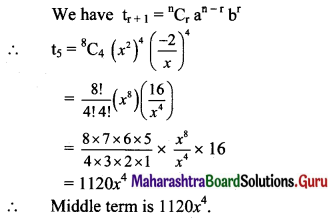(iv) $$\left(\frac{x}{a}-\frac{a}{x}\right)^{10}$$
Solution: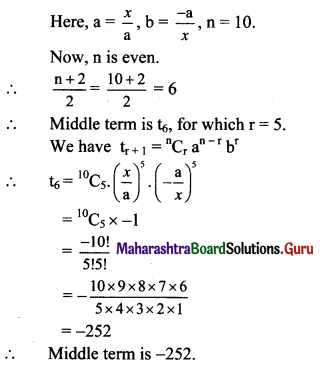(v) $$\left(x^{4}-\frac{1}{x^{3}}\right)^{11}$$
Solution: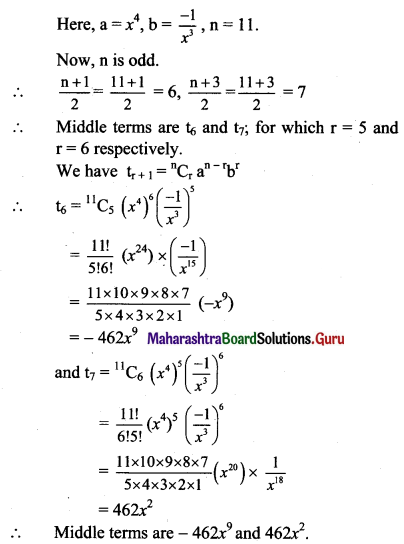Question 5.
In the expansion of (k + x)8, the coefficient of x5 is 10 times the coefficient of x6. Find the value of k.
Solution: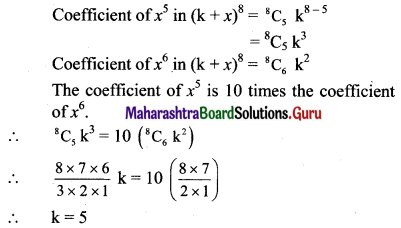Question 6.
Find the term containing x6 in the expansion of (2 – x) (3x + 1)9.
Solution: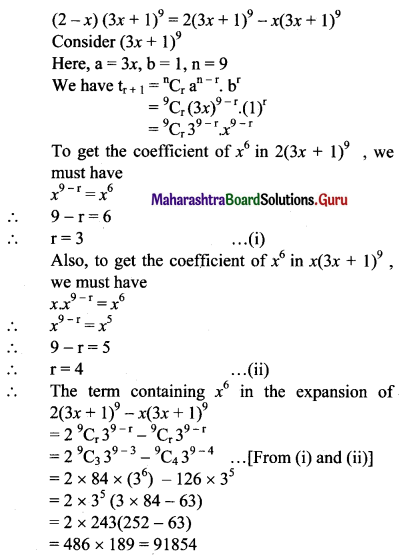Question 7.
The coefficient of x2 in the expansion of (1 + 2x)m is 112. Find m.
Solution:
The coefficient of x2 in (1 + 2x)m = mC2 (22)
Given that the coefficient of x2 = 112
mC2 (4) = 112
mC2 = 28
∴ $$\frac{\mathrm{m} !}{2 !(\mathrm{m}-2) !}=28$$
∴ $$\frac{m(m-1)(m-2) !}{2 \times(m-2) !}=28$$
∴ m(m – 1) = 56
∴ m2 – m – 56 = 0
∴ (m – 8) (m + 7) = 0
As m cannot be negative.
∴ m = 8